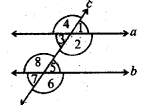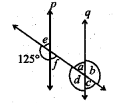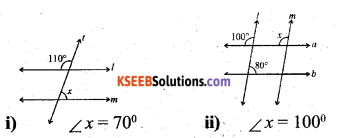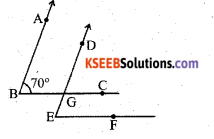# KSEEB Solutions for Class 7 Maths Chapter 5 Lines and Angles Ex 5.2

Students can Download Chapter 5 Lines and Angles Ex 5.2, Question and Answers, Notes Pdf, KSEEB Solutions for Class 7 Maths, Karnataka State Board Solutions help you to revise complete Syllabus and score more marks in your examinations.

## Karnataka State Syllabus Class 7 Maths Chapter 5 Lines and Angles Ex 5.2

Question 1.
State the property that is used in each of the following statements ?i) If a || b, then ∠1 = ∠5
Solution:
The two parallel lines a & b are cut by a transversal then ∠1 = ∠5 because they are corresponding angles. So they have equal measure.

ii) If ∠4 = ∠6, then a || b.
Solution:
If ∠4 and ∠6 are equal measures they are interior alternate angles. So. a is parallel to b.

iii) If ∠4 + ∠5 = 180°, then a || b.
Solution:
Here two parallel lines are cut by transversal, ∠4 and ∠5 are pairs of interior angles on the same side of the transversal are supplementary.Question 2.i) the pairs of corresponding angles.
Solution:
There are 4 pairs of corresponding angles,
a) ∠1 and ∠5
b) ∠2 and ∠6
c) ∠4 and ∠8
d) ∠3 and ∠7

ii) the pairs of alternate interior angles.
Solution:
There are 2 pairs of alternate interior angles. They are
a) ∠3 and ∠5
b) ∠2 and ∠8

iii) the pairs of interior angles on the same side of the transversal.
Solution:
There are 2 pairs interior angles on the same side of the transversal, they are
a) ∠3 and ∠8
b) ∠2 and ∠5

iv) the vertically opposite angles.
Solution:
There are 4 pairs of vertically opposite angles. They are
a) ∠1 and ∠3
b) ∠2 and ∠4
c) ∠5 and ∠7
d) ∠6 and ∠8.Question 3.
In the adjoining figure, p || q. Find the unknown angles.Solution:
The unknown angles are
∠e = 180° – 125° = 55° (supplementary angles)
∠f = ∠e = 55° (∵ vertically opposite angles)
∠a = ∠e = 55° ∠b = 125°
∠c = ∠a = 55° (∵ vertically opposite angles)
∠d = ∠b = 125°Question 4.
Find the value of x in each of the following figures if l || m.
Solution:Question 5.
In the given figure, the arms of two angles are parallel. If ∠ABC = 70°, then find
Solution:i) ∠DGC
∠DGC = ∠ABC = 70°
ii) ∠DEF
∠DEF = ∠ABC = 70°Question 6.
In given figures below, decide whether l is parallel to m.
i)l is not parallel to m. ( ∵ the sum of the angles are not supplementary. 126 + 44 = 170 ≠ 180)

ii)l is not parallel to m

iii)>
l is parallel to m
(∵ 123 + 57 = 180)

iv) l is not parallel to m.error: Content is protected !!